2022: SklogWiki celebrates 15 years on-line

# Isothermal-isobaric ensemble

The isothermal-isobaric ensemble has the following variables:

•$N$ is the number of particles
•$p$ is the pressure
•$T$ is the temperature

The classical partition function, for a one-component atomic system in 3-dimensional space, is given by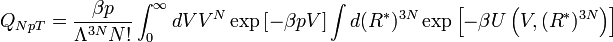$Q_{NpT} = \frac{\beta p}{\Lambda^{3N} N!} \int_{0}^{\infty} d V V^{N} \exp \left[ - \beta p V \right] \int d ( R^*)^{3N} \exp \left[ - \beta U \left(V,(R^*)^{3N} \right) \right]$

where

•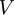$\left. V \right.$ is the Volume:
•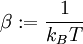$\beta := \frac{1}{k_B T}$, where$k_B$ is the Boltzmann constant
•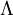$\left. \Lambda \right.$ is the de Broglie thermal wavelength
•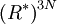$\left( R^* \right)^{3N}$ represent the reduced position coordinates of the particles; i.e.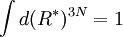$\int d ( R^*)^{3N} = 1$
•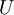$\left. U \right.$ is the potential energy, which is a function of the coordinates (or of the volume and the reduced coordinates)